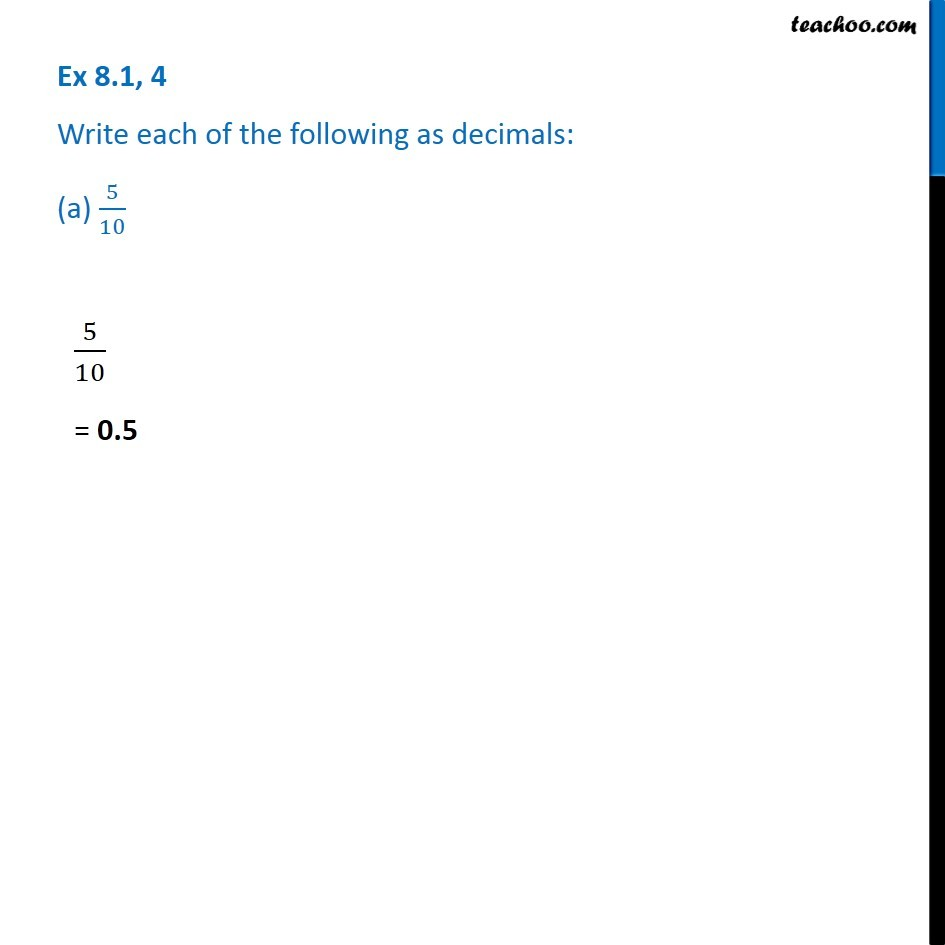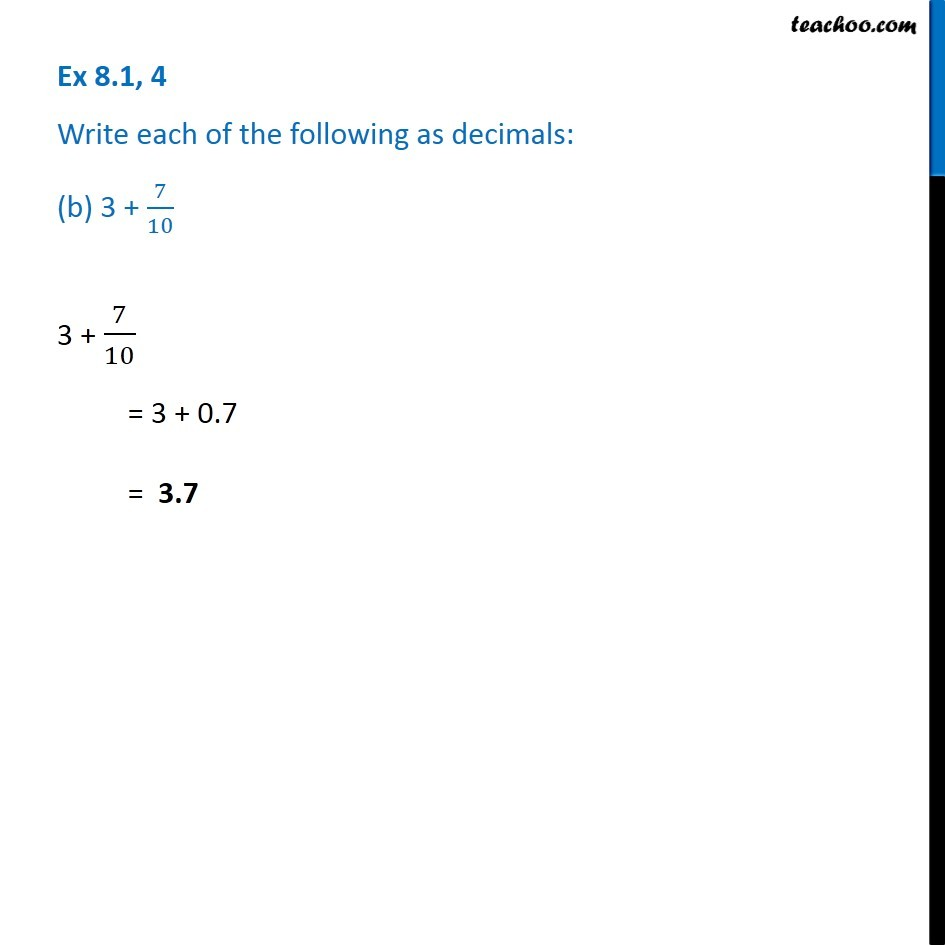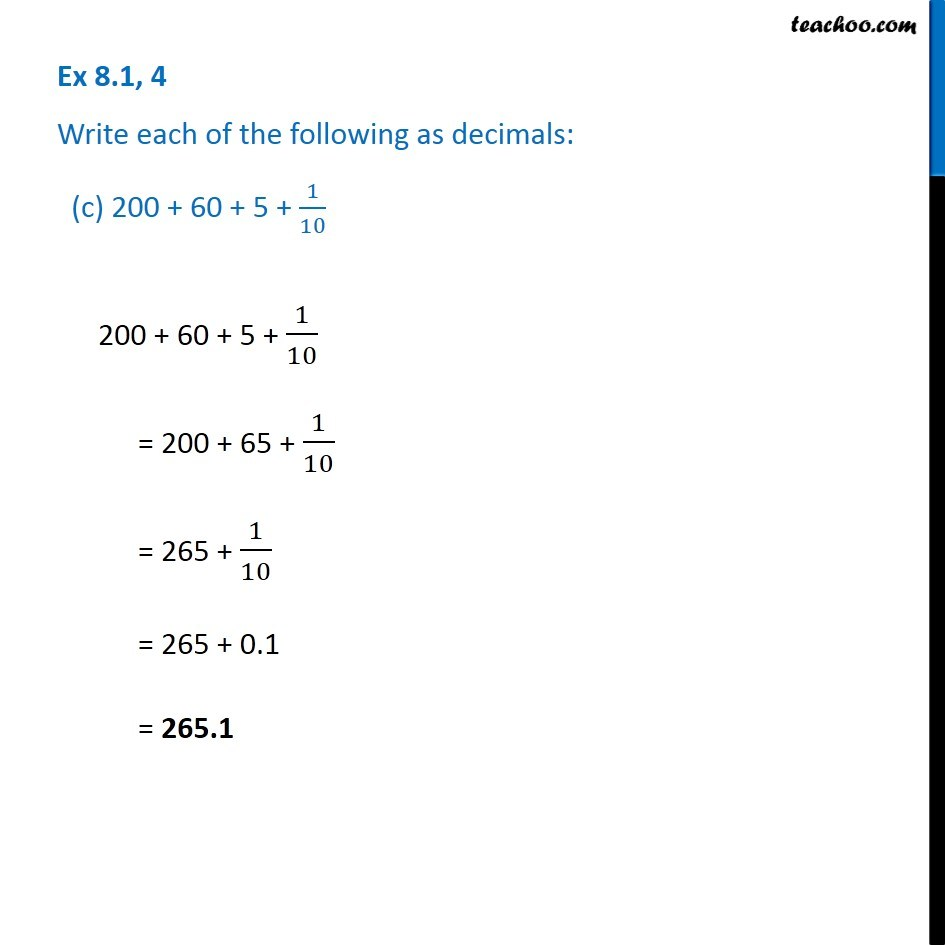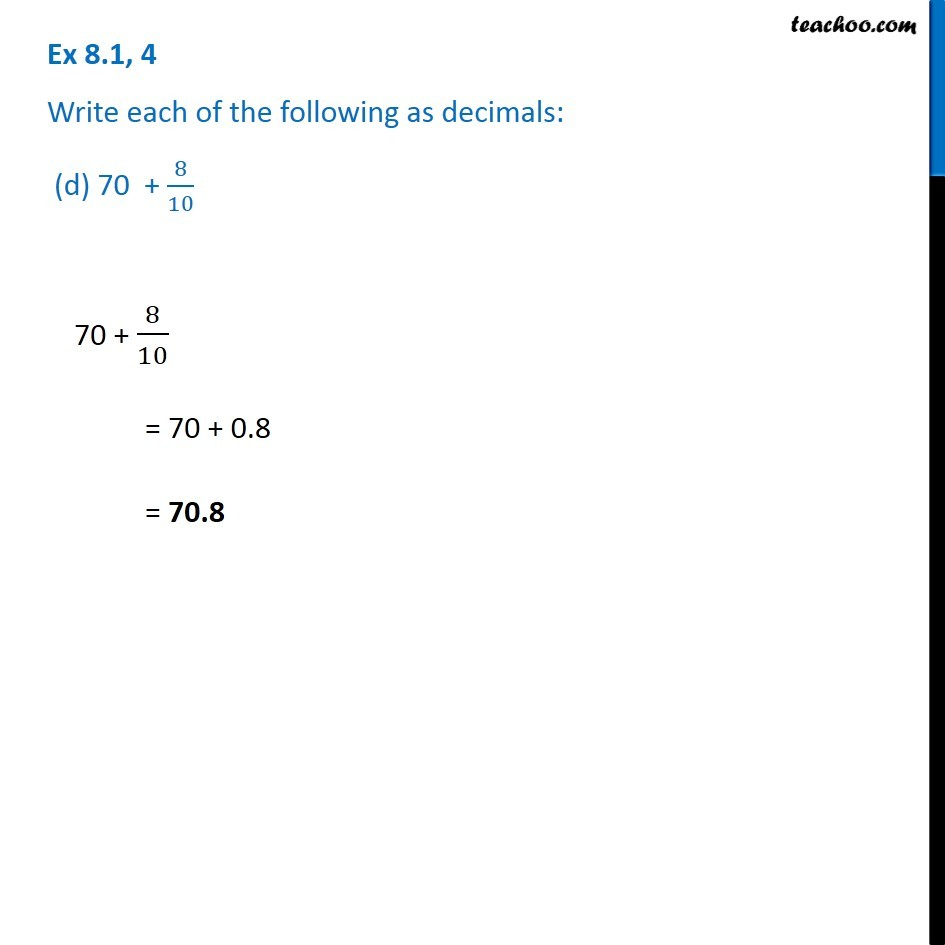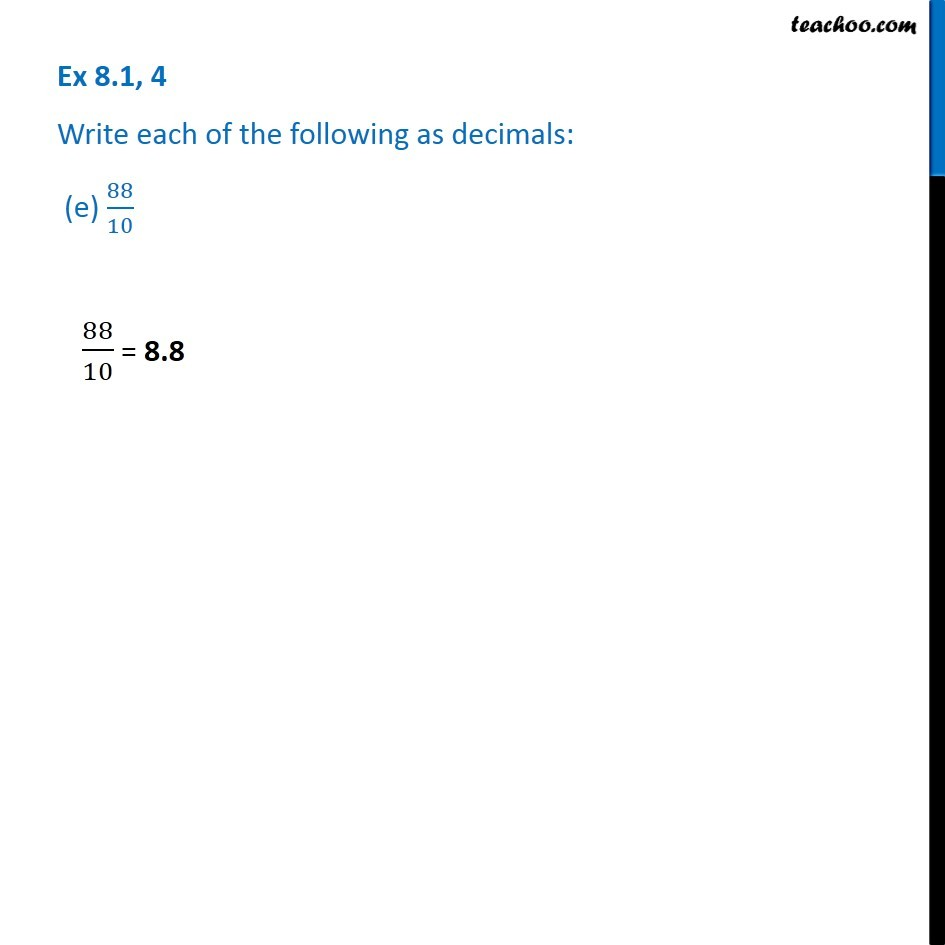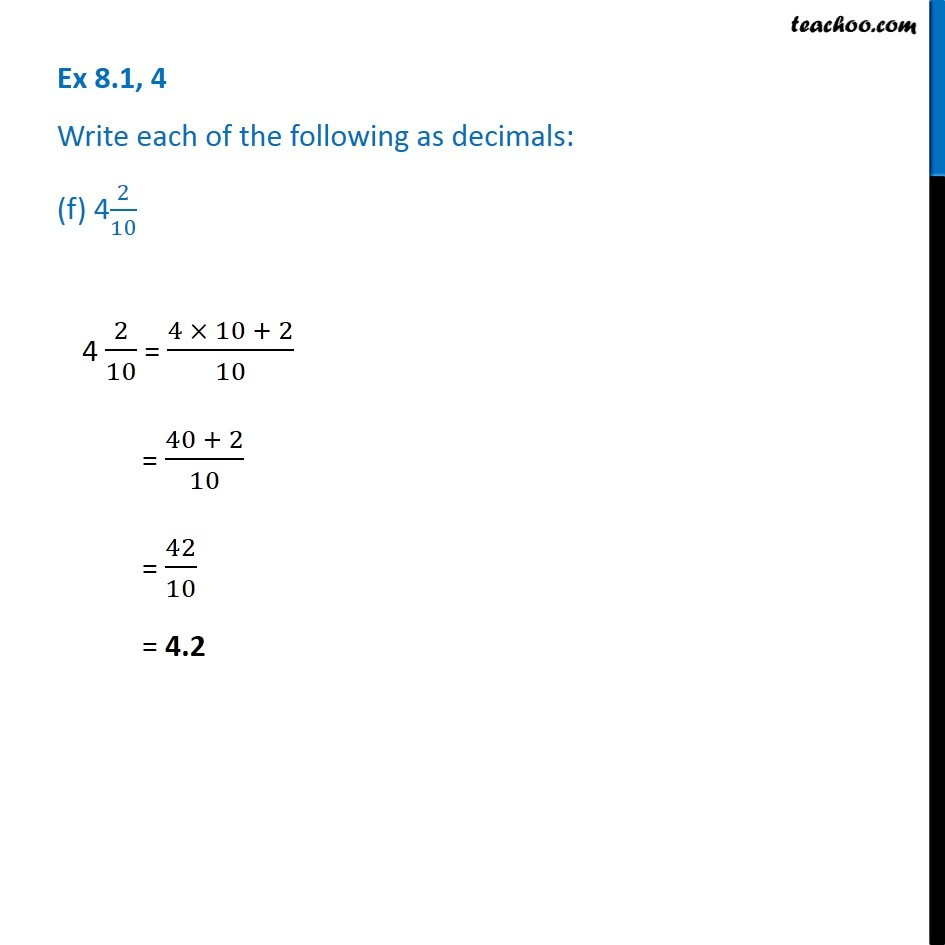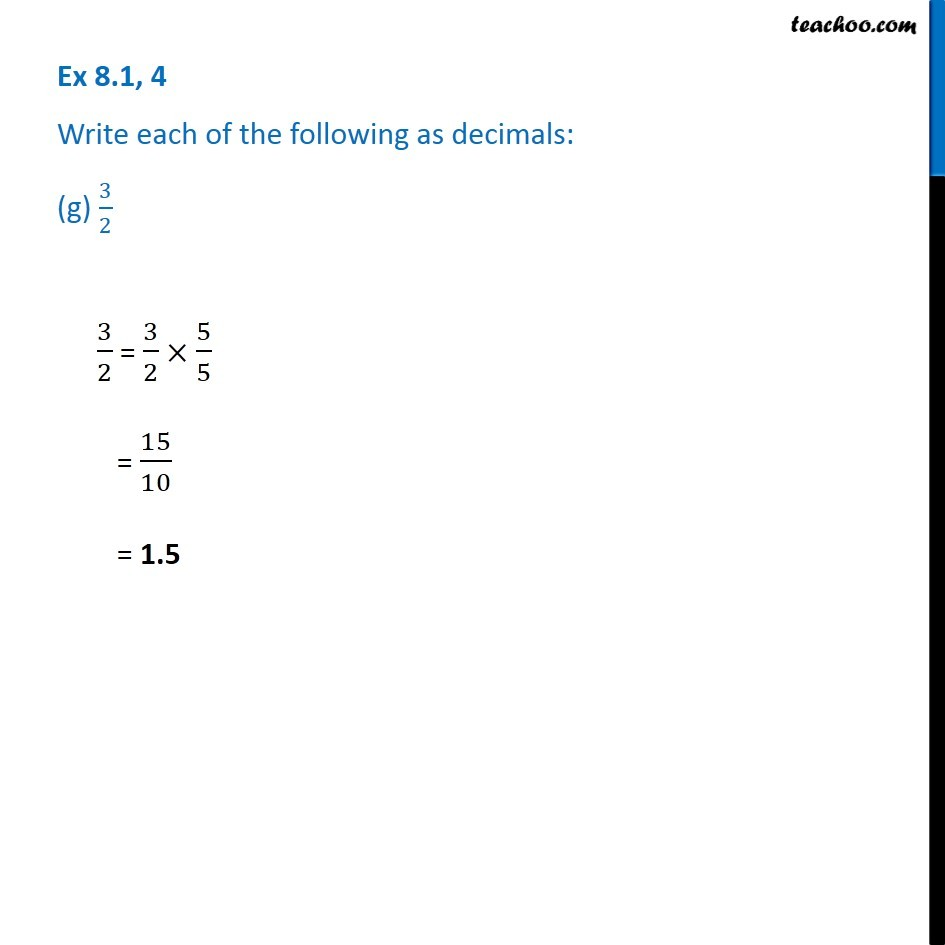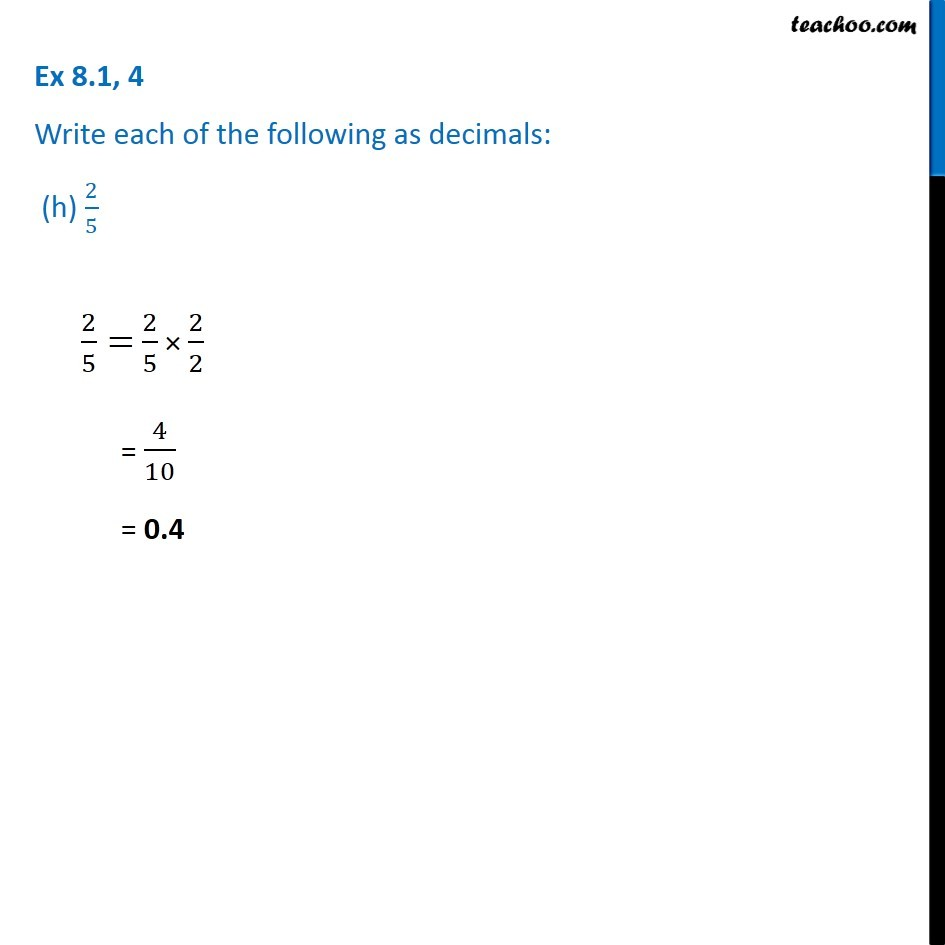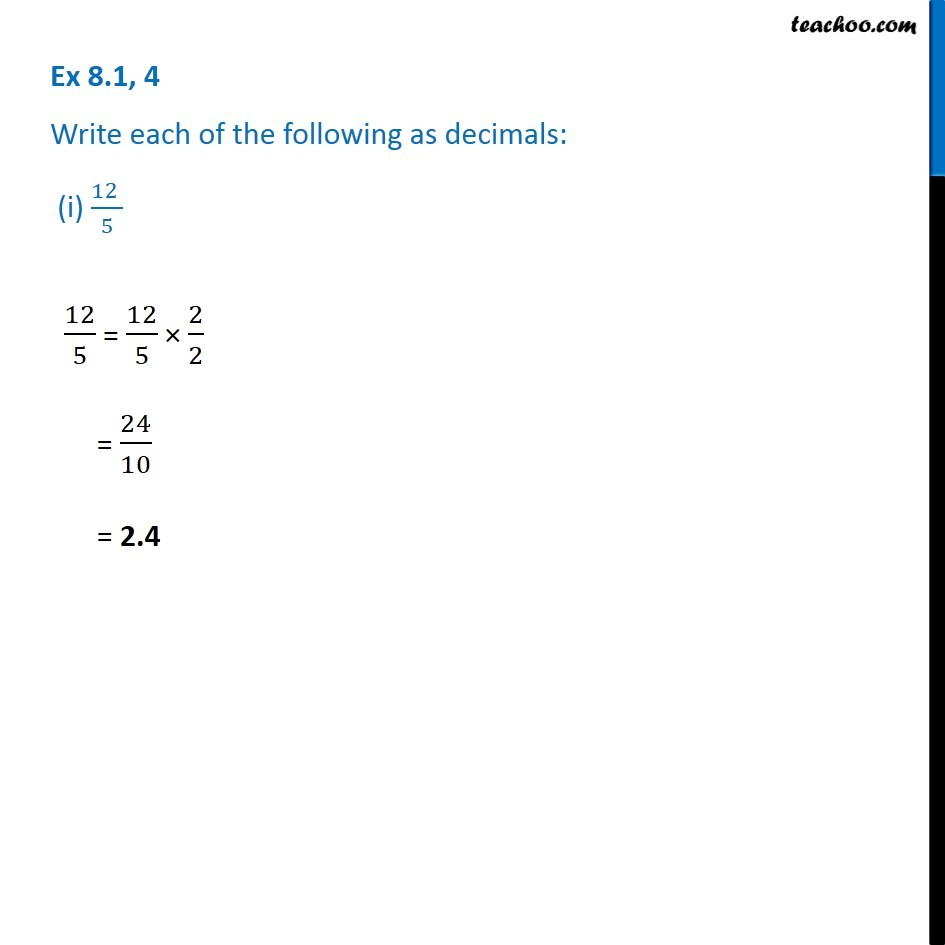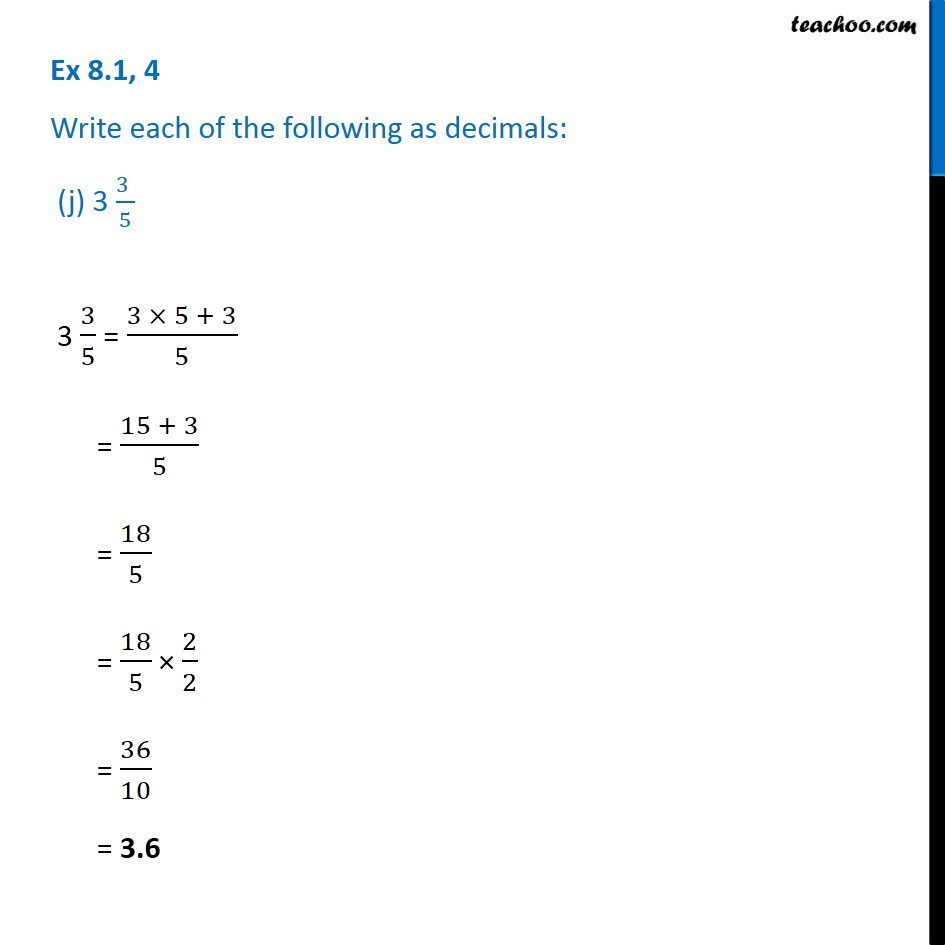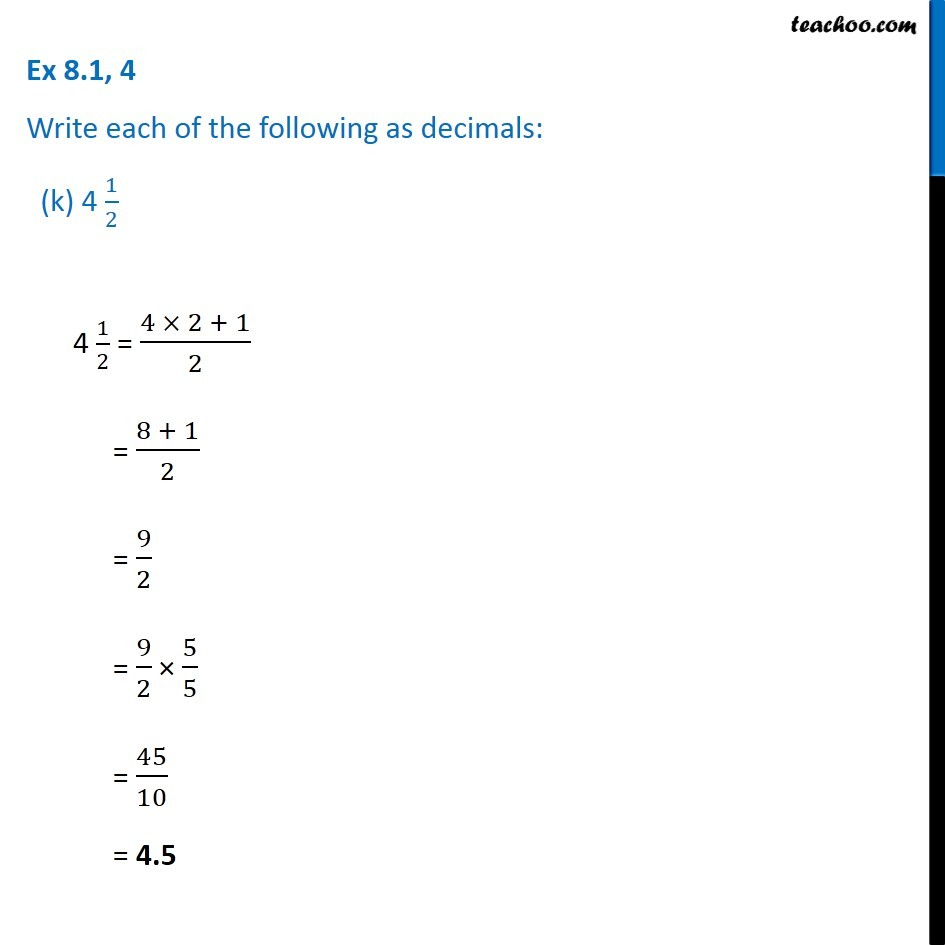Subscribe to our Youtube Channel - https://you.tube/teachoo

1. Chapter 8 Class 6 Decimals
2. Serial order wise
3. Ex 8.1

Transcript

Ex 8.1, 4 Write each of the following as decimals: (a) 5/10 5/10 = 0.5 Ex 8.1, 4 Write each of the following as decimals: (b) 3 + 7/10 3 + 7/10 = 3 + 0.7 = 3.7 Ex 8.1, 4 Write each of the following as decimals: (c) 200 + 60 + 5 + 1/10 200 + 60 + 5 + 1/10 = 200 + 65 + 1/10 = 265 + 1/10 = 265 + 0.1 = 265.1 Ex 8.1, 4 Write each of the following as decimals: (d) 70 + 8/1070 + 8/10 = 70 + 0.8 = 70.8 Ex 8.1, 4 Write each of the following as decimals: (e) 88/10 88/10 = 8.8 Ex 8.1, 4 Write each of the following as decimals: (f) 42/104 2/10 = (4 × 10 + 2)/10 = (40 + 2)/10 = 42/10 = 4.2 Ex 8.1, 4 Write each of the following as decimals: (g) 3/2 3/2 = 3/2 × 5/5 = 15/10 = 1.5 Ex 8.1, 4 Write each of the following as decimals: (h) 2/5 Ex 8.1, 4 Write each of the following as decimals: (h) 2/5 2/5= 2/5 × 2/2 = 4/10 = 0.4Ex 8.1, 4 Write each of the following as decimals: (i) (12 )/5 12/5 = 12/5 × 2/2 = 24/10 = 2.4 Ex 8.1, 4 Write each of the following as decimals: (j) 3 (3 )/5 3 3/5 = (3 × 5 + 3)/5 = (15 + 3)/5 = 18/5 = 18/5 × 2/2 = 36/10 = 3.6 Ex 8.1, 4 Write each of the following as decimals: (k) 4 1/2 4 1/2 = (4 × 2 + 1)/2 = (8 + 1)/2 = 9/2 = 9/2 × 5/5 = 45/10 = 4.5

Ex 8.1

Chapter 8 Class 6 Decimals
Serial order wise